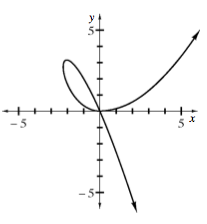### Home > CALC > Chapter 6 > Lesson 6.4.1 > Problem6-131

6-131.
1. The graph of x3 = 5xy + 2y2 is shown below. Homework Help ✎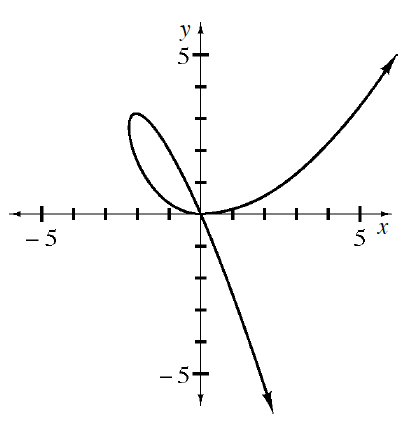1. How many tangent lines exist at x = 2?

2. Find the equations of the tangent lines at x = −2.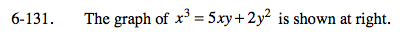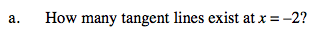How many coordinate points does this graph have at
x = −2?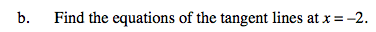Write two tangent line equations in point-slope form.